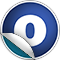# Iterator

#### object Iterator

The `Iterator` object provides various functions for creating specialized iterators.

Version

2.8

Since

2.8

Linear Supertypes
AnyRef, Any
Ordering
1. Alphabetic
2. By inheritance
Inherited
1. Iterator
2. AnyRef
3. Any
1. Hide All
2. Show all
Visibility
1. Public
2. All

### Value Members

1. #### final def !=(arg0: Any): Boolean

Definition Classes
AnyRef → Any
2. #### final def ##(): Int

Definition Classes
AnyRef → Any
3. #### final def ==(arg0: Any): Boolean

Definition Classes
AnyRef → Any
4. #### implicit def IteratorCanBuildFrom[A]: BufferedCanBuildFrom[A, Iterator]

With the advent of `TraversableOnce` and `Iterator`, it can be useful to have a builder which operates on `Iterator`s so they can be treated uniformly along with the collections.

With the advent of `TraversableOnce` and `Iterator`, it can be useful to have a builder which operates on `Iterator`s so they can be treated uniformly along with the collections. See `scala.util.Random.shuffle` for an example.

5. #### def apply[A](elems: A*): Iterator[A]

Creates an iterator with given elements.

Creates an iterator with given elements.

elems

The elements returned one-by-one from the iterator

returns

An iterator which produces the given elements on the first calls to `next`, and which has no further elements.

6. #### final def asInstanceOf[T0]: T0

Definition Classes
Any
7. #### def clone(): AnyRef

Attributes
protected[java.lang]
Definition Classes
AnyRef
Annotations
( ... )
8. #### def continually[A](elem: ⇒ A): Iterator[A]

Creates an infinite-length iterator returning the results of evaluating an expression.

Creates an infinite-length iterator returning the results of evaluating an expression. The expression is recomputed for every element.

elem

the element computation.

returns

the iterator containing an infinite number of results of evaluating `elem`.

9. #### val empty: Iterator[Nothing]

The iterator which produces no values.

10. #### final def eq(arg0: AnyRef): Boolean

Definition Classes
AnyRef
11. #### def equals(arg0: Any): Boolean

Definition Classes
AnyRef → Any
12. #### def fill[A](len: Int)(elem: ⇒ A): Iterator[A]

Creates iterator that produces the results of some element computation a number of times.

Creates iterator that produces the results of some element computation a number of times.

len

the number of elements returned by the iterator.

elem

the element computation

returns

An iterator that produces the results of `n` evaluations of `elem`.

13. #### def finalize(): Unit

Attributes
protected[java.lang]
Definition Classes
AnyRef
Annotations
( classOf[java.lang.Throwable] )
14. #### def from(start: Int, step: Int): Iterator[Int]

Creates an infinite-length iterator returning values equally spaced apart.

Creates an infinite-length iterator returning values equally spaced apart.

start

the start value of the iterator

step

the increment between successive values

returns

the iterator producing the infinite sequence of values `start, start + 1 * step, start + 2 * step, ...`

15. #### def from(start: Int): Iterator[Int]

Creates an infinite-length iterator which returns successive values from some start value.

Creates an infinite-length iterator which returns successive values from some start value.

start

the start value of the iterator

returns

the iterator producing the infinite sequence of values `start, start + 1, start + 2, ...`

16. #### final def getClass(): Class[_]

Definition Classes
AnyRef → Any
17. #### def hashCode(): Int

Definition Classes
AnyRef → Any
18. #### final def isInstanceOf[T0]: Boolean

Definition Classes
Any
19. #### def iterate[T](start: T)(f: (T) ⇒ T): Iterator[T]

Creates an infinite iterator that repeatedly applies a given function to the previous result.

Creates an infinite iterator that repeatedly applies a given function to the previous result.

start

the start value of the iterator

f

the function that's repeatedly applied

returns

the iterator producing the infinite sequence of values `start, f(start), f(f(start)), ...`

20. #### final def ne(arg0: AnyRef): Boolean

Definition Classes
AnyRef
21. #### final def notify(): Unit

Definition Classes
AnyRef
22. #### final def notifyAll(): Unit

Definition Classes
AnyRef
23. #### def range(start: Int, end: Int, step: Int): Iterator[Int]

An iterator producing equally spaced values in some integer interval.

An iterator producing equally spaced values in some integer interval.

start

the start value of the iterator

end

the end value of the iterator (the first value NOT returned)

step

the increment value of the iterator (must be positive or negative)

returns

the iterator producing values `start, start + step, ...` up to, but excluding `end`

24. #### def range(start: Int, end: Int): Iterator[Int]

Creates nn iterator returning successive values in some integer interval.

Creates nn iterator returning successive values in some integer interval.

start

the start value of the iterator

end

the end value of the iterator (the first value NOT returned)

returns

the iterator producing values `start, start + 1, ..., end - 1`

25. #### def single[A](elem: A): Iterator[A]

Creates an iterator which produces a single element.

Creates an iterator which produces a single element. Note: Equivalent, but more efficient than Iterator(elem)

elem

the element

returns

An iterator which produces `elem` on the first call to `next`, and which has no further elements.

26. #### final def synchronized[T0](arg0: ⇒ T0): T0

Definition Classes
AnyRef
27. #### def tabulate[A](end: Int)(f: (Int) ⇒ A): Iterator[A]

Creates an iterator producing the values of a given function over a range of integer values starting from 0.

Creates an iterator producing the values of a given function over a range of integer values starting from 0.

end

The number of elements returned by the iterator

f

The function computing element values

returns

An iterator that produces the values `f(0), ..., f(n -1)`.

28. #### def toString(): String

Definition Classes
AnyRef → Any
29. #### final def wait(): Unit

Definition Classes
AnyRef
Annotations
( ... )
30. #### final def wait(arg0: Long, arg1: Int): Unit

Definition Classes
AnyRef
Annotations
( ... )
31. #### final def wait(arg0: Long): Unit

Definition Classes
AnyRef
Annotations
( ... )